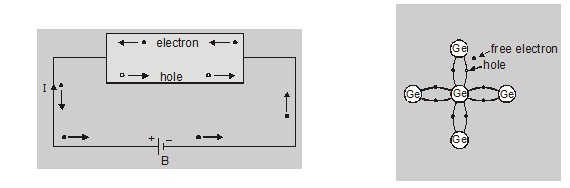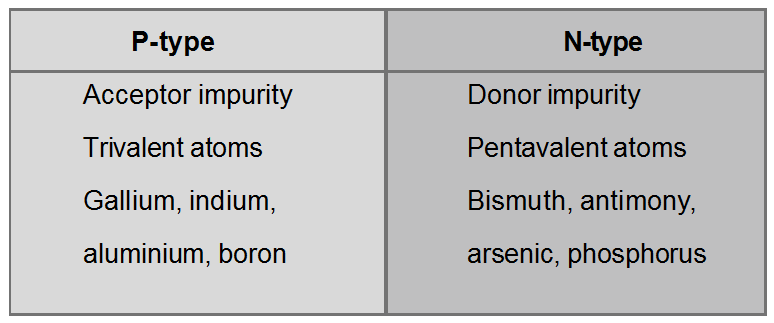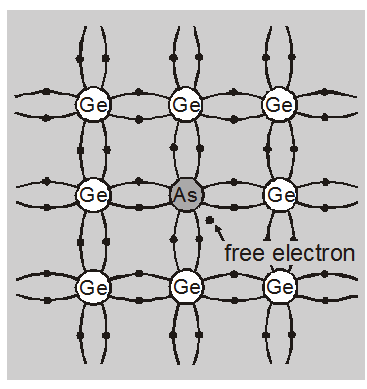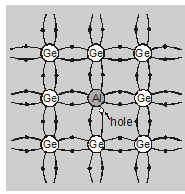Most Affordable JEE | NEET | 8,9,10 Preparation by Kota's Top IITian Doctor Faculties

# Types of Semiconductors - Types, Examples - eSaral`

Semiconductor in an extremely pure form is known as an intrinsic semiconductor and The impure semiconductor material is called an extrinsic semiconductor. If you want to learn about the Types of Semiconductors then keep reading.

## Types of Semiconductors

### Pure or intrinsic semiconductor:

A semiconductor in an extremely pure form is known as an intrinsic semiconductor. In an intrinsic semiconductor, the number of free electrons is always equal to the number of holes when an external field is applied across the intrinsic semiconductor the conduction through the semiconductor is by both free electrons and holes.• Total current $I=I_{e}+I_{h}$

• It is perfectly neutral

• Number of free electron = Number of holes $\left(n_{e}=n_{h}\right)$

### Extrinsic semiconductor:

The intrinsic semiconductors are of little importance because of negligible conductivity and moreover, the conductivity has little flexibility.

The electrical conductivity of an intrinsic semiconductor is zero at absolute zero and very small at ordinary (room) temperatures.

The conductivity is considerably increased by adding some impurity element to the pure (intrinsic) semiconductors.

The impure semiconductor material is called an extrinsic semiconductor.

The electrical conductivity of extrinsic semiconductors is called extrinsic conductivity.

Depending on impurity added, the extrinsic semiconductor can be divided into two parts-N-Type:

An n-type Ge is obtained by adding a small quantity, for $10^{6}$ Ge atoms approximately one impurity atom of a pentavalent impurity

added the ratio of Ge and As atoms is $10^{6}: 1$.

Generally, Arsenic (As) is taken for this purpose.Each arsenic atom replaces one Ge atom at its crystal lattice site without changing its structure. Four of the five valence electrons of As occupy the same positions in four covalent bonds as earlier occupied by four electrons of replaced Ge atom. But the fifth excess electron remains free. The energy level of this excess electron is only slightly smaller than the lowest energy level of the conduction band. Very small energy of about 0.01 eV (0.05 eV for Si) can detach this electron from the impurity atom.

The thermal energy at room temperature is sufficient to enable this electron to detach itself and become a member of the conduction band and take part in conduction.

Why n-type

Electrons with negative charges help in current conduction, the impure Ge is called n-type. Since impurity atoms donate electrons, the impurity is called donor impurity.

Each $1 \mathrm{~cm}^{3}$ of Ge crystal having $4.52 \times 10^{22}$ Ge atom, will have $4.52 \times 10^{16}$ (one millionth) As atoms and hence as many free electrons. The conductivity of the crystal is increased considerably.

At high temperatures, some covalent bonds are broken. Then more electrons and holes become free.

Free holes in n-type Ge act as minority carriers.

• $\mathrm{n}_{\mathrm{e}}$$>>$$\mathrm{n}_{\mathrm{h}}$

• $\mathrm{I}=\mathrm{I}_{\mathrm{e}}+\mathrm{I}_{\mathrm{h}}$

$\left(\mathrm{I}_{\mathrm{e}}>>\mathrm{I}_{\mathrm{h}}\right)$ $I \approx I_{e}$

In N-Type semiconductor current mainly flow due to free electrons.

• Energy needed to detach fifth electron from impurity for 0.01 eV for Ge, 0.05 eV for Si.

• Electrons are majority carriers due to the addition of pentavalent impurity.

• Holes are minority carriers due to the breaking of covalent bonds.

• N-type semiconductor has an excess of free electrons but it is electrically neutral.

• It is called donor-type impurity because it gives one electron to a crystal.

• The type of conductivity is called negative or N-type conductivity.

#### P-type:

A p-type Ge is obtained by adding a small quantity for $10^{6}$ Ge atoms approximately one impurity atom of a trivalent impurity added. Ratio of Ge and Al atoms is $10^{6}: 1$.Generally, Aluminum (Al) or Boron (B) is taken for this purpose.

Each aluminum atom replaces one Ge atom at its crystal lattice site without changing its structure. The three valence electrons of Al occupy the same positions in three covalent bounds as earlier occupied by four electrons of replaced Ge atom.

One covalent bond remains unfilled which shown an electron vacancy.

This electron vacancy is called a hole. The energy of this hole is slightly more than the highest energy level of the valence band (figure).

The electrons from the valence band get easily excited by thermal energy at room temperature to enter the hole. But the electrons filling these holes create new holes in the valence band. These holes in the valence band are filled by more electrons and this continues.

Holes can move freely through a crystal lattice and take part in conduction.

Why p-type

Since holes with positive charges help in current conduction, the impure Ge is called p-type. Since impurity atoms accept electrons (for their holes), the impurity is called acceptor impurity. Each $1 \mathrm{~cm}^{3}$ of Ge crystal having $4.52 \times 10^{22}$ Ge many holes. The conductivity of the crystal is increased considerably.

At high temperatures, some covalent bonds are broken. Then more electrons and holes become free.

Free electrons in p-type Ge act as minority carriers.

• $n_{h}>>n_{e}$

• $\mathrm{I}=\mathrm{I}_{\mathrm{e}}+\mathrm{I}_{\mathrm{h}}$

$\left(\mathrm{I}_{\mathrm{h}}>>\mathrm{I}_{\mathrm{e}}\right)$
$\mathrm{I} \approx \mathrm{I}_{\mathrm{h}}$

In p-type semiconductor current mainly flow due to holes.

• In a p-type semiconductor, majority carriers are positive holes due to the addition of trivalent impurity.

• In a p-type semiconductor, minority carriers are electrons due to the breaking of covalent bonds.

• This impurity is called acceptor type impurity

• In P-type, the valence electrons move from one covalent bond to another bond.